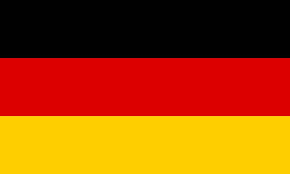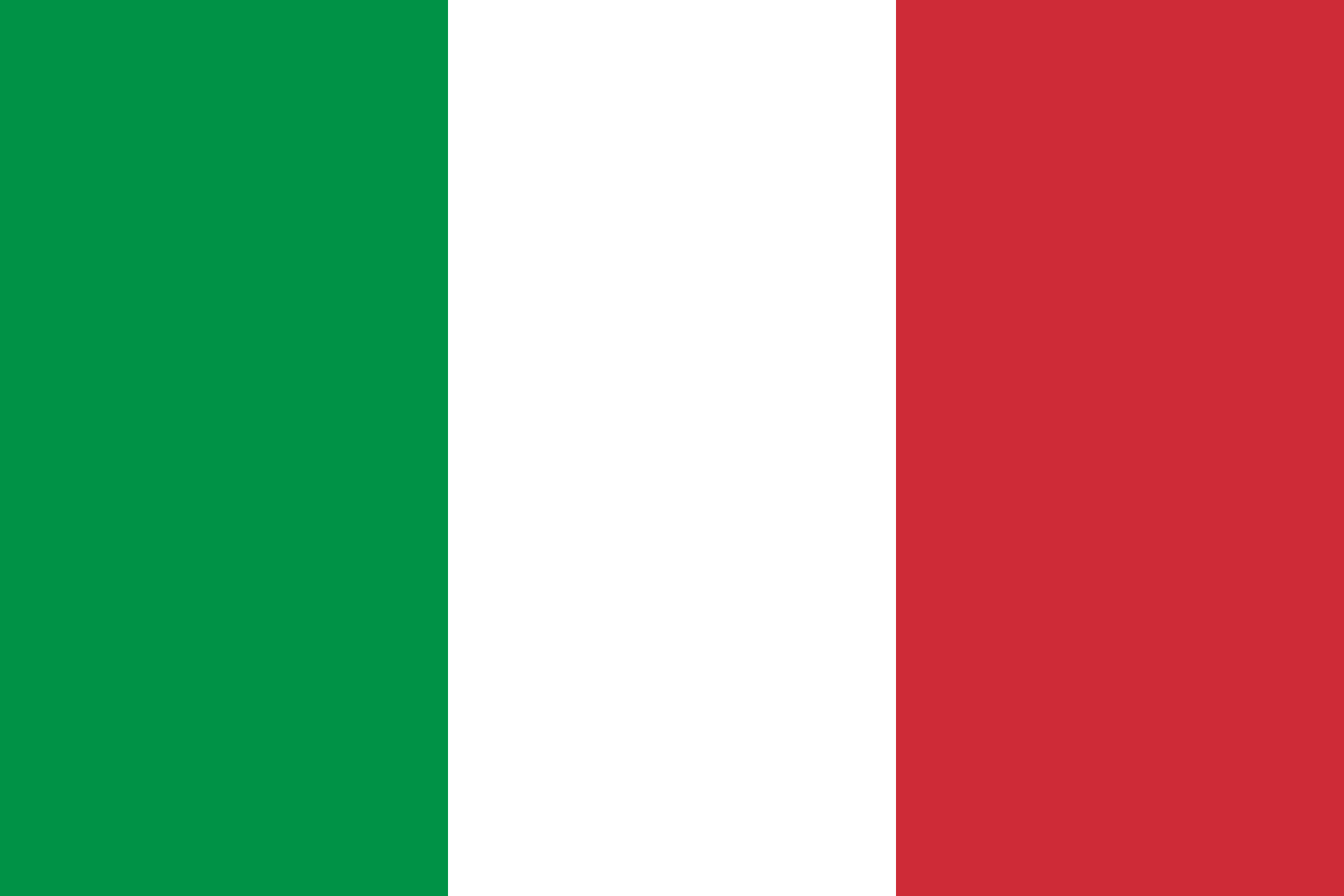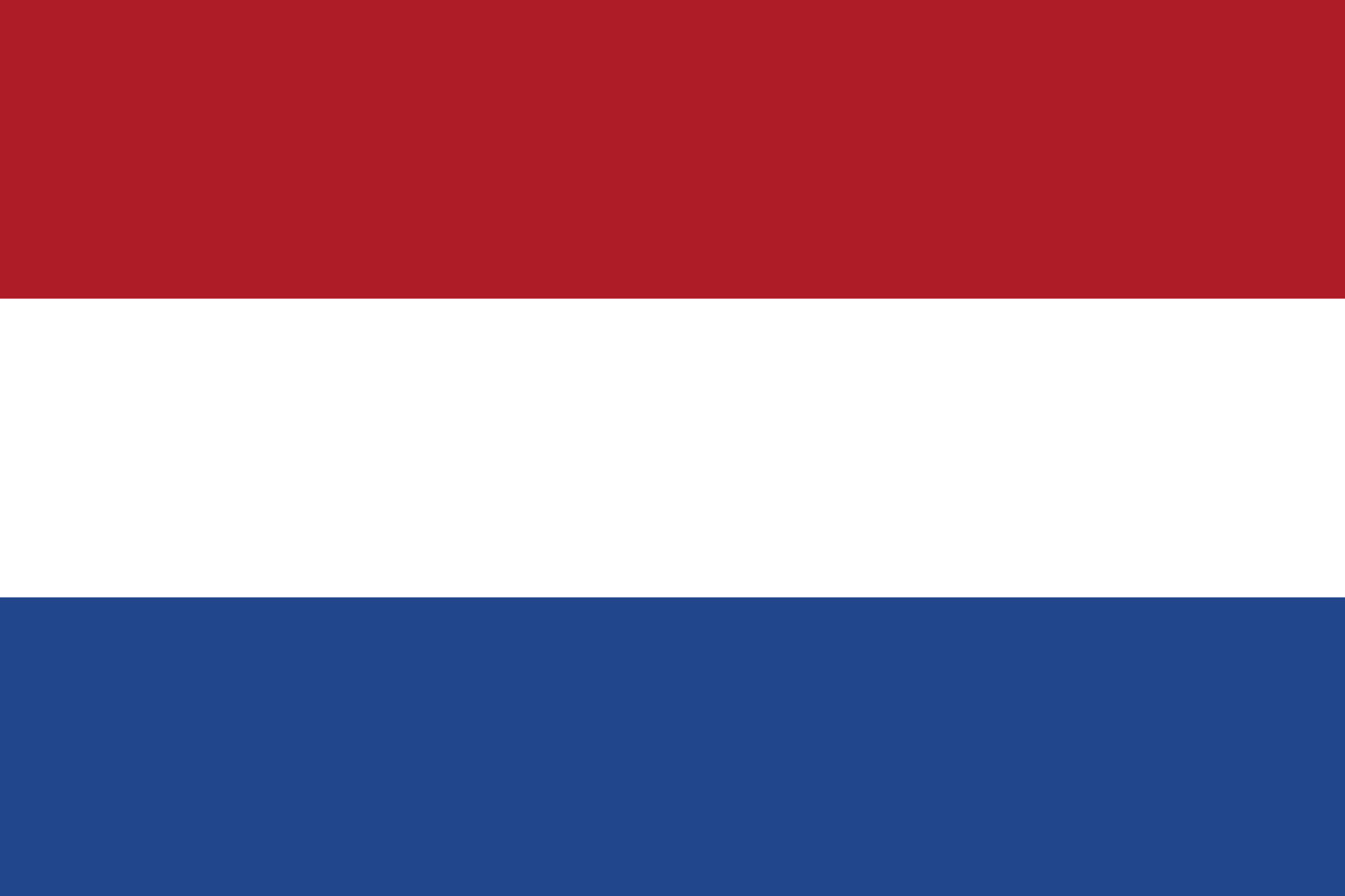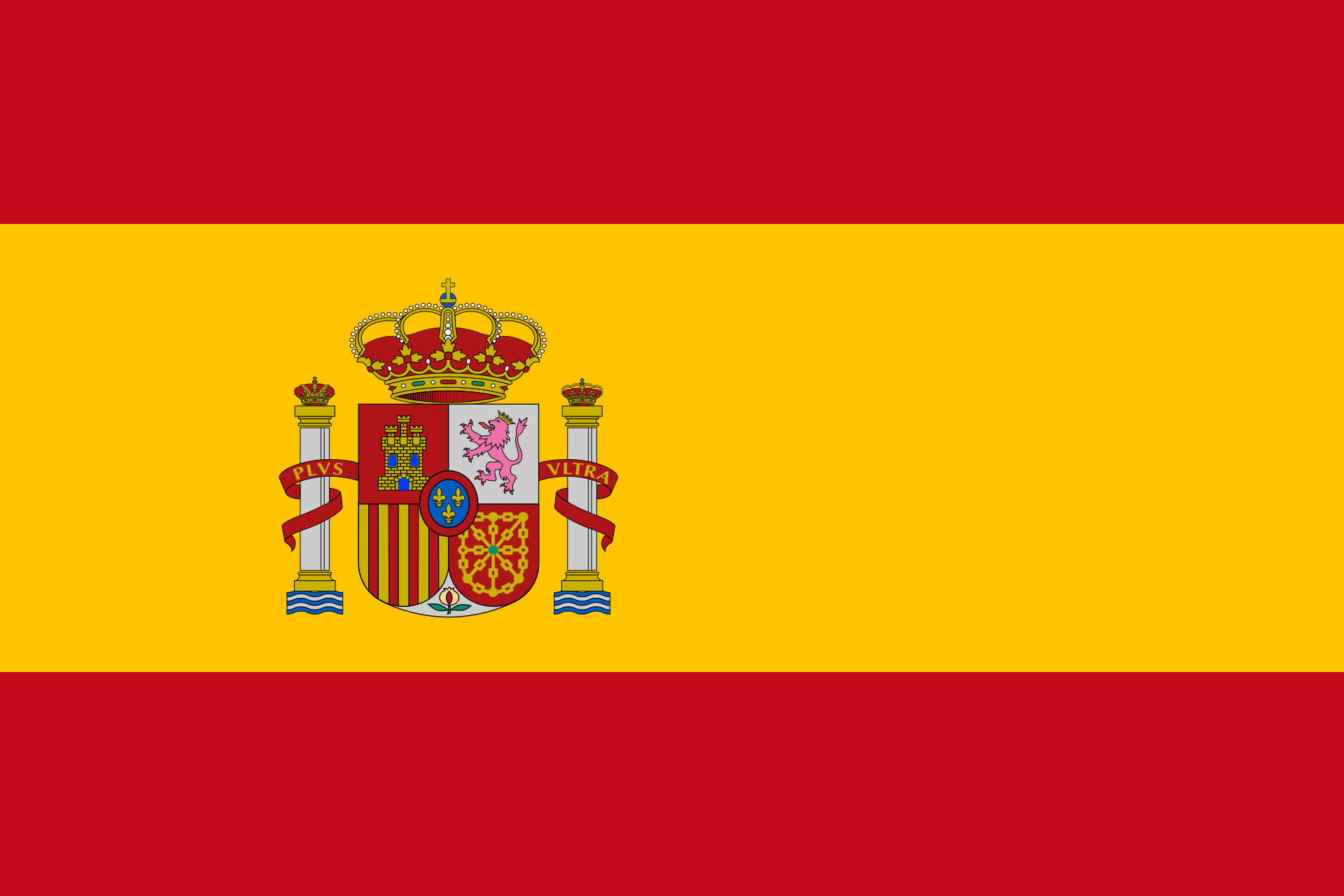# Point on planeEnter a point and a plane. Mathepower checks if the point is on the plane.

P(||)

Plane

Choose how the plane is given.
• parametric equation:

 E: x =+ r+ s• Coordinate form:

• Point-normal form:

 E: (x-)⋅=0
• Given through three points

## How to check if a point is on a plane?

Simply insert the point into one of the plane equations and solve the corresponding system of equations.